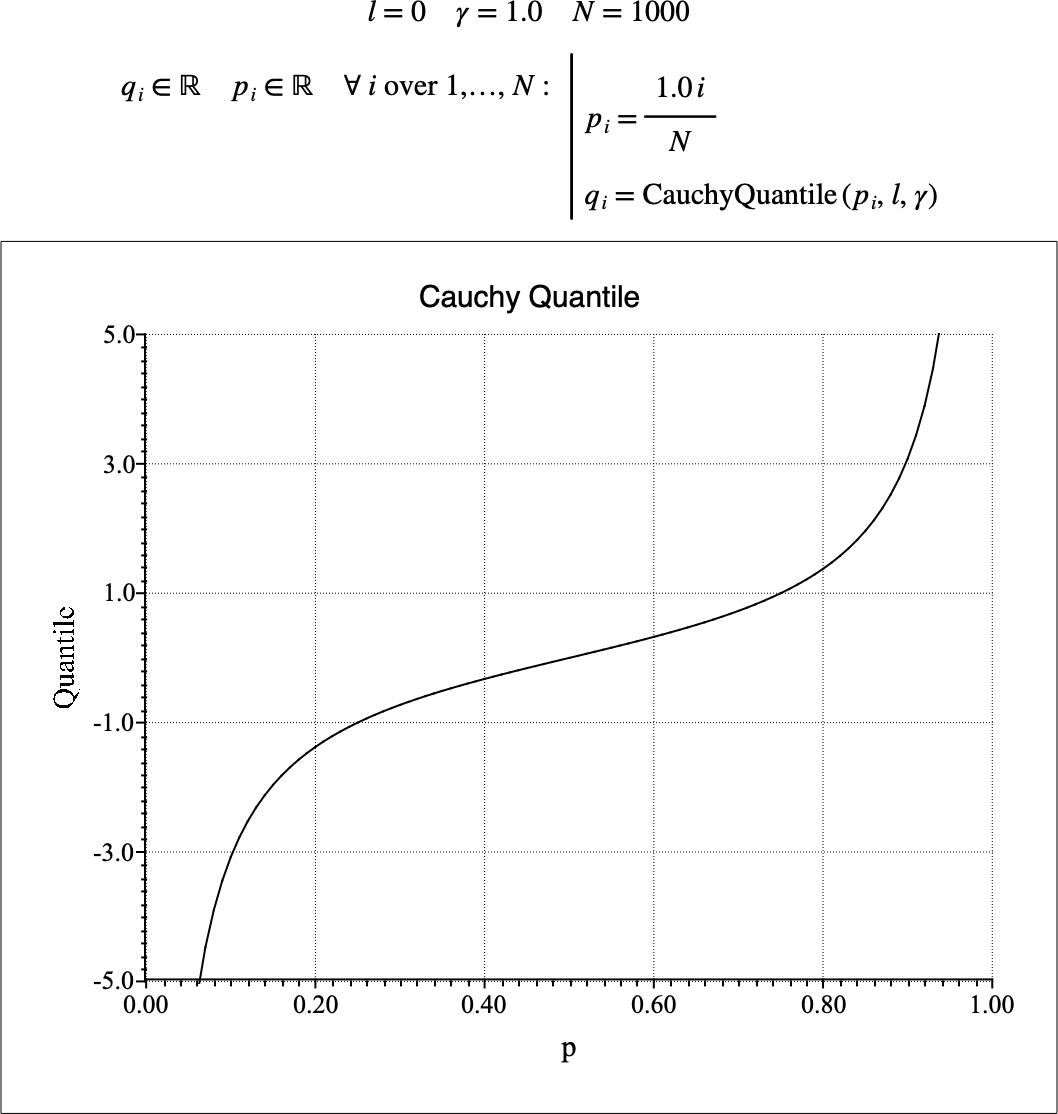# $$\text{CauchyQuantile}$$¶

You can use the $$\text{CauchyQuantile}$$ function to calculate the quantile function of the Cauchy-Lorentz distribution. The quantile function is the inverse of the cumulative distribution function.

You can use the \cauchyq backslash command to insert this function.

The following variants of this function are available:

• $$\text{real } \text{CauchyQuantile} \left ( \text{<p>}, \text{<location>}, \text{<}\gamma\text{>} \right )$$

Where $$p$$, $$location$$, and $$\gamma$$ are scalar values representing the probability, the location or offset ($$x _ 0$$), and the scale term respectively. Note that this function is defined over the range $$\gamma > 0$$ and $$0 \leq p \leq 1$$ and will generate a runtime error or return NaN for values for which the function is not defined.

The value is calculated directly using the relation:

$\text{CauchyQuantile} \left ( p, l, \gamma \right ) = x _ 0 + \gamma \tan \left [ \pi \left ( p - \frac{1}{2} \right ) \right ]$

Figure 114 shows the basic use of the $$\text{CauchyQuantile}$$ function.Figure 114 Example Use Of the CauchyQuantile Function